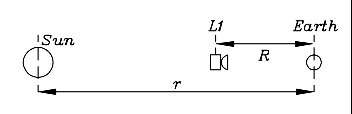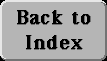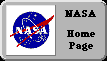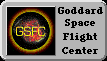# (34a)The Distance to the L1 Point

By using the tools of sections (20) and (21), the mathematics of approximate solutions developed in (M-5), and assuming all orbits are circles, it is a relatively straightforward job to calculate the distance to the Lagrangian L1 point (or to L2). We start by repeating the derivation of Kepler's 3rd law for circular orbits.
Note:The formats available on the world-wide web require that all fractions be represented by slashes. It may be a good idea to copy them out (as you come to them) on a sheet of paper, using conventional fraction bars, which will give them a more familiar form.Let the gravitational constant be denoted by G, the mass of the Sun by M, that of Earth by m, the Earth's distance from the Sun by r and its velocity in its orbit by v. By applying what was learned about gravity and about centripetal and centrifugal forces, we then get (as in section (20))

GMm/ r2  =  mv2/ r

and multiplying both sides by r/ m

GM/ r  =  v2

If T is the orbital period, since the distance covered by the Earth each orbit is 2pr,

vT = 2p r
Divide by T
v = 2p r/ T
Square:
v2 = 4p2r2/ T2
and therefore
GM/ r  = 4p2r2/ T2
Divide both sides by r2:
GM/ r3  = 4p2/ T2

from which Kepler's 3rd law for circular orbits follows (just multiply both sides by r3T2).

Consider next a spacecraft of mass ms c , located on the line connecting the Earth and the Sun, between those two at a distance R from Earth and (r-R) from the Sun (drawing). The force F pulling it sunward is decreased by the pull of the Earth in the opposite direction, so

F = GMms c / (r-R)2 - Gmms c / R2

Assume that this spacecraft also moves in a circle around the Sun, with velocity vsc. Then if the centrifugal force balances the attraction (or else, the attraction supplies the centripetal force)

GMms c / (r-R)2 - Gmms c / R2  =  ms c vs c 2/ (r-R)

In a similar way to the earlier calculation, both sides are multiplied by (r-R)/ ms c , giving

GM/ (r-R) - Gm(r-R)/ R2  =  (vs c )2

We assume that the spacecraft also moves in a circle around the Sun, of radius (r-R). (That of course requires the Earth to be always in place to pull in the opposite direction from the Sun, a subject to which we will return shortly). The orbital period Ts c  of the satellite then satisfies, as before

vs c Ts c  = 2p(r-R)
from which
(vs c )2 = 4p2(r-R)2/ (Ts c )2
We then have

GM/ (r-R) - Gm(r-R)/ R2  =  4p2(r-R)2/ (Ts c )2

or, dividing everything by (r-R)2

GM/ (r-R)3 - Gm/ R2(r-R)  =  4p2/ (Ts c )2

which resembles the earlier "3rd law" equation, except that the Earth's opposing pull is now added.But will the Earth be always located where its pull on the satellite is exactly opposite to the Sun? No, unless the two orbital periods are the same:

Ts c  = T

Only then does the spacecraft's motion match that of the Earth and the distance between the two stays constant. That in general only happens at one value of R, that is, only at one distance from the Earth, and that distance, R, is now the unknown number we need to derive, the "thing" that we seek. If Tsc  = T, the two relations with 4p2/T2 on the right side are equal to each other, thus providing an equation from which R can be derived. That equation is

GM/ (r-R)3 - Gm/ R2(r-R)  =  GM/ r3

Let us divide both sides by GM: then G disappears from the scene, and in place of the masses (m,M) of (Earth,Sun) we only have their ratio, a small number which we will name y

y = m/ M = 3/ 1,000,000

or in scientific notation (the dot meaning multiplication)

y = m/ M = 3/ 106   (or 3.10-6)

The equation is now

1/ (r-R)3 - y/ R2(r-R)  =  1/ r3

This can be further simplified. Instead of working with both r and R, let us introduce their ratio z = R/ r, which is also a small number, since the spacecraft is always much closer to Earth than to the Sun. To do so, multiply both sides by r3, which can also be achieved by dividing denominators on both sides by r3. The result:

1/ (1-z)3 - y/ z2(1-z) = 1

Now we are ready to roll, to derive the unknown quantity z. The above equation is complicated--in fact, an exact formula for its solution probably does not exist. However, since z is small, it is rather easy to get an approximate solution, using the methods of section (M-5). There is was shown that

1/ (1-z)3  ~  1 + 3z
and
[y/ z2]/ (1-z)  ~  [y/ z2] (1+z)

Substituting these approximations in the main equation gives

1 + 3z - [y/ z2] (1+z)  ~  1
From this
3z  ~  [y/ z2] (1+z)
and hence
3z3  ~  y(1+z)

Both sides are now equally small. The right-hand side is only slightly modified by the addition of z: we may drop this term without making much difference. Then

3z3  ~  y  =  3/ 1,000,000

z3  ~  1/ 1,000,000
Take 3rd root of both sides
R/ r = z  ~  1/ 100 = 0.01

Thus the distance to L1 is about 0.01 of the distance to the Sun. It is now possible to go back to more exact equations, like 3z3 ~ y(1+z), and replace z on the right by its approximate value 0.01, to get more accurate solutions. Such a process of gradual improvement, called iteration, is also used with Kepler's equation, discussed in section (12a). Because of the smallness of z, these modifications turn out here to make very little difference.

The derivation of the distance to the nightside Lagrangian point L2 is very similar (as is its result). In that case the equations involve r+R rather than r-R, and in the equation for F the terms are added instead of subtracted, because now the Sun and the Earth pull in the same direction. Try it!

Optional:  #34b  The L4 and L5 Lagrangian Points

Next Stop:  #35   To the Planets, to the StarsAuthor and curator: David P. Stern, u5dps@lepvax.gsfc.nasa.gov
Last updated 15 January 1999